# An Introduction on Time Series Forecasting with Simple Neura Networks & LSTM

Source: Deep Learning on MediumPhoto Credit: Pixabay

## How to develop Artificial Neural Networks and LSTM recurrent neural networks for time series prediction in Python with the Keras deep learning network

The purpose of this article is to explain Artificial Neural Network (ANN) and Long Short-Term Memory Recurrent Neural Network (LSTM RNN) and enable you to use them in real life and build the simplest ANN and LSTM recurrent neural network for the time series data.

### The Data

The CBOE Volatility Index, known by its ticker symbol VIX, is a popular measure of the stock market’s expectation of volatility implied by S&P 500 index options. It is calculated and disseminated on a real-time basis by the Chicago Board Options Exchange (CBOE).

The VOLATILITY S&P 500 data set can be downloaded from here, I set the date range from Feb 11, 2011 to Feb 11, 2019. Our goal is to predict VOLATILITY S&P 500 time series using ANN & LSTM.

First, we will need to import the following libraries:

`import pandas as pdimport numpy as np%matplotlib inlineimport matplotlib.pyplot as pltfrom sklearn.preprocessing import MinMaxScalerfrom sklearn.metrics import r2_scorefrom keras.models import Sequentialfrom keras.layers import Densefrom keras.callbacks import EarlyStoppingfrom keras.optimizers import Adamfrom keras.layers import LSTM`

And load the data into a Pandas dataframe.

`df = pd.read_csv("vix_2011_2019.csv")`

We can have a quick peek of the first few rows.

`print(df.head())`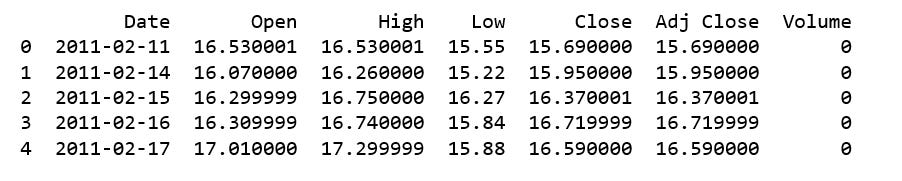Figure 1

We will drop the columns we don’t need, then convert “Date” column to datatime data type and set “Date” column to index.

`df.drop(['Open', 'High', 'Low', 'Close', 'Volume'], axis=1, inplace=True)df['Date'] = pd.to_datetime(df['Date'])ind_df = df.set_index(['Date'], drop=True)ind_df.head()`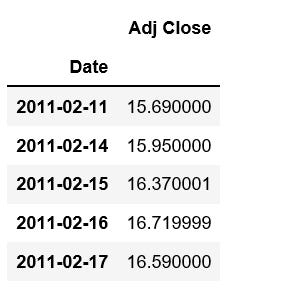Figure 2

Next, we plot a time series line plot.

`ind_df = ind_df.sort_index()plt.figure(figsize=(10, 6))ind_df['Adj Close'].plot();`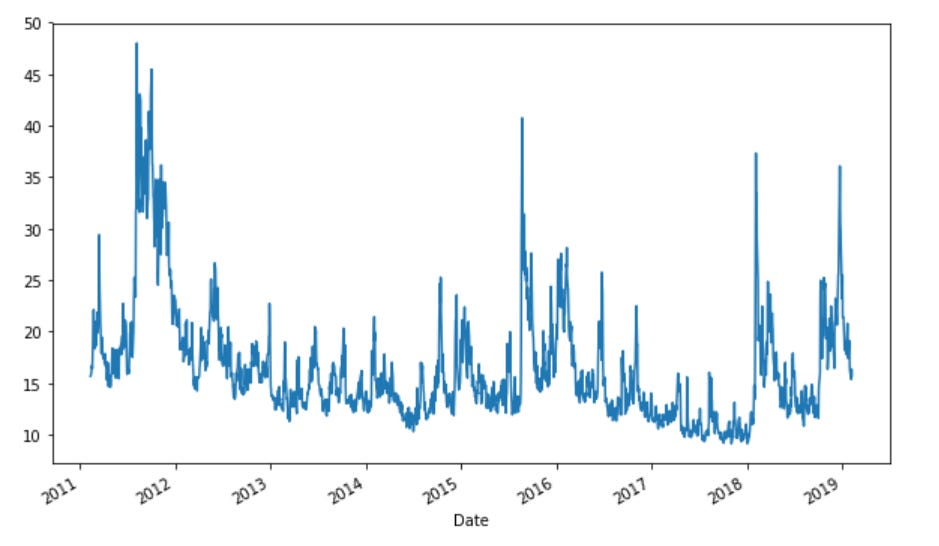Figure 3

As can be seen, the “Adj close” data are quite erratic, seems neither upward trend nor downward trend.

We split the data to train and test set by date “2018–01–01”, that is, the data prior to this date is the training data and the data from this data onward is the test data, and we visualize it again.

`split_date = pd.Timestamp('2018-01-01')df =  df['Adj Close']train = df.loc[:split_date]test = df.loc[split_date:]plt.figure(figsize=(10, 6))ax = train.plot()test.plot(ax=ax)plt.legend(['train', 'test']);`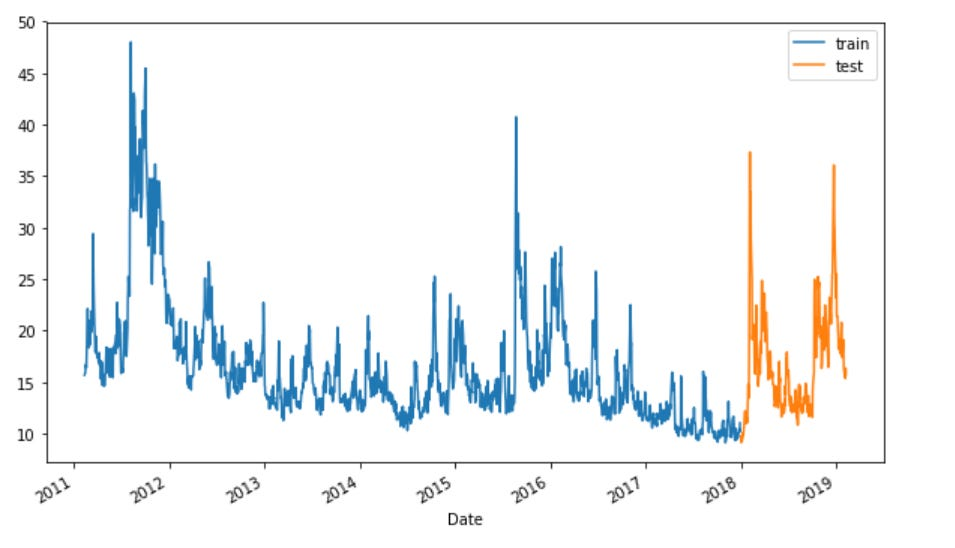Figure 4

We scale train and test data to [-1, 1].

`scaler = MinMaxScaler(feature_range=(-1, 1))train_sc = scaler.fit_transform(train)test_sc = scaler.transform(test)`

Shuffle the training set, but do not shuffle the test set.

`np.random.shuffle(train_sc)`

Get training and test data.

`X_train = train_sc[:-1]y_train = train_sc[1:]`
`X_test = test_sc[:-1]y_test = test_sc[1:]`

### Simple ANN for Time Series Forecasting

• We create a `Sequential` model.
• add layers via the `.add()` method.
• Pass an `input_dim` argument to the first layer.
• The activation function is the Rectified Linear Unit- Relu.
• Configure the learning process, which is done via the `compile` method.
• A loss function is `mean_squared_error` , and An optimizer is `adam`.
• Stop training when a monitored `loss` has stopped improving.
• `patience=2`, indicate number of epochs with no improvement after which training will be stopped.
• The ANN is trained for 100 epochs and a batch size of 1 is used.
`nn_model = Sequential()nn_model.add(Dense(12, input_dim=1, activation='relu'))nn_model.add(Dense(1))nn_model.compile(loss='mean_squared_error', optimizer='adam')early_stop = EarlyStopping(monitor='loss', patience=2, verbose=1)history = nn_model.fit(X_train, y_train, epochs=100, batch_size=1, verbose=1, callbacks=[early_stop], shuffle=False)`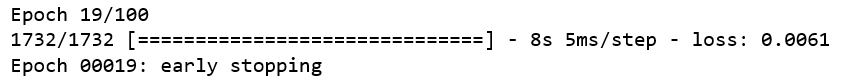Figure 5

I will not print out the entire output. It had an early stopping at Epoch 19/100.

`y_pred_test_nn = nn_model.predict(X_test)y_train_pred_nn = nn_model.predict(X_train)print("The R2 score on the Train set is:\t{:0.3f}".format(r2_score(y_train, y_train_pred_nn)))print("The R2 score on the Test set is:\t{:0.3f}".format(r2_score(y_test, y_pred_test_nn)))`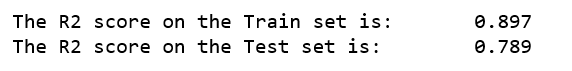Figure 6

### LSTM

When constructing LSTM, we will use pandas `shift` function that shifts the entire column by 1. In the below code snippet, we shifted the column down by 1. Then we will need to convert all our input variables to be represented in a 3D vector form.construct_LSTM.py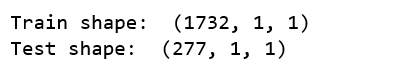Figure 7

The LSTM networks creation and model compiling is similar with those of ANN’s.

• The LSTM has a visible layer with 1 input.
• A hidden layer with 7 LSTM neurons.
• An output layer that makes a single value prediction.
• The `relu` activation function is used for the LSTM neurons.
• The LSTM is trained for 100 epochs and a batch size of 1 is used.
`lstm_model = Sequential()lstm_model.add(LSTM(7, input_shape=(1, X_train_lmse.shape), activation='relu', kernel_initializer='lecun_uniform', return_sequences=False))lstm_model.add(Dense(1))lstm_model.compile(loss='mean_squared_error', optimizer='adam')early_stop = EarlyStopping(monitor='loss', patience=2, verbose=1)history_lstm_model = lstm_model.fit(X_train_lmse, y_train, epochs=100, batch_size=1, verbose=1, shuffle=False, callbacks=[early_stop])`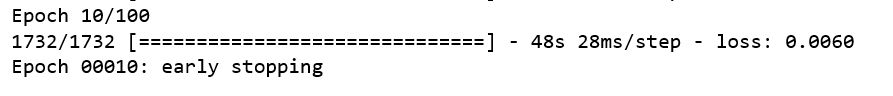Figure 8

It had an early stopping at Epoch 10/100.

`y_pred_test_lstm = lstm_model.predict(X_test_lmse)y_train_pred_lstm = lstm_model.predict(X_train_lmse)print("The R2 score on the Train set is:\t{:0.3f}".format(r2_score(y_train, y_train_pred_lstm)))print("The R2 score on the Test set is:\t{:0.3f}".format(r2_score(y_test, y_pred_test_lstm)))`Figure 9

Both training and test R^2 are better than those of ANN model.

### Compare Models

We compare test MSE of both models.

`nn_test_mse = nn_model.evaluate(X_test, y_test, batch_size=1)lstm_test_mse = lstm_model.evaluate(X_test_lmse, y_test, batch_size=1)print('NN: %f'%nn_test_mse)print('LSTM: %f'%lstm_test_mse)`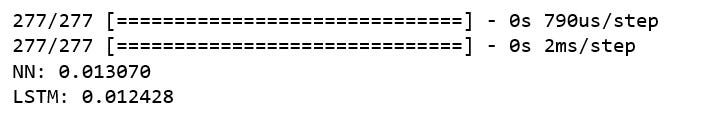Figure 10

### Making Predictions

`nn_y_pred_test = nn_model.predict(X_test)lstm_y_pred_test = lstm_model.predict(X_test_lmse)`
`plt.figure(figsize=(10, 6))plt.plot(y_test, label='True')plt.plot(y_pred_test_nn, label='NN')plt.title("NN's Prediction")plt.xlabel('Observation')plt.ylabel('Adj Close Scaled')plt.legend()plt.show();`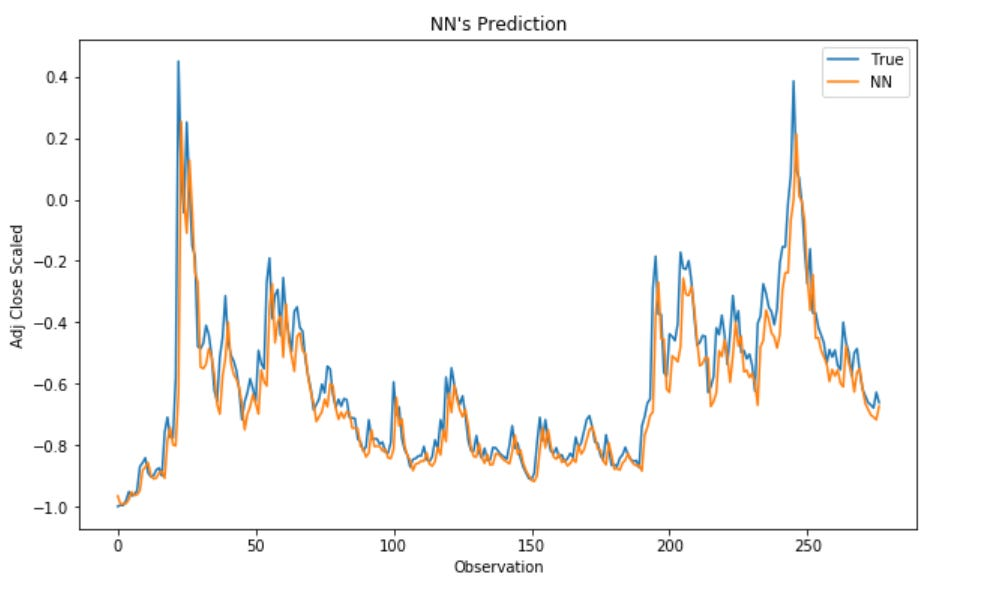Figure 11
`plt.figure(figsize=(10, 6))plt.plot(y_test, label='True')plt.plot(y_pred_test_lstm, label='LSTM')plt.title("LSTM's Prediction")plt.xlabel('Observation')plt.ylabel('Adj Close scaled')plt.legend()plt.show();`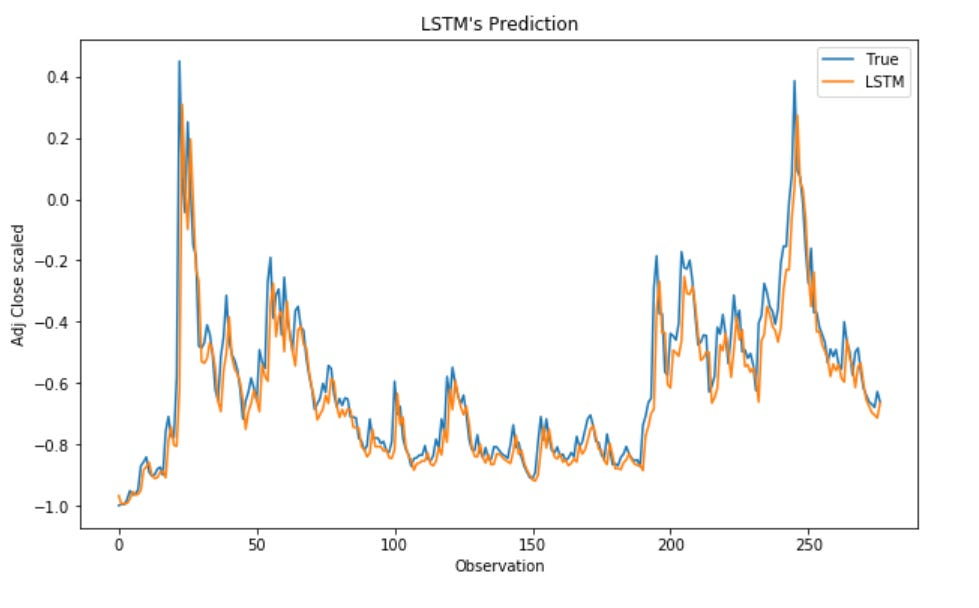Figure 12

That was it! In this post, we have discovered how to develop ANN and LSTM recurrent neural networks for time series prediction in Python with the Keras deep learning network, and how can they be leveraged to better predict time series data.

Jupyter notebook can be found on Github. Happy Monday!

Reference: Keras documentation